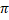# CBSE Solutions for Class 10 Maths

#### Select CBSE Solutions for class 10 Subject & Chapters Wise :

What is the diameter of the circle whose area is equal to the sum of the areas of two circles having radii 4 cm and 3 cm?What is the area of a circle whose circumference is 8π?What is the perimeter of a semicircular protractor whose diameter is 14 cm?What is the radius of a circle whose perimeter and area are numerically equal?Circumference of a circle is 39.6 cm. find its area.

Circumference of circle = 2 πr = 39.6 cmCircumference of a circle is 22 cm. Find the area of its quadrant.A circle whose area is equal to the sum of the areas of two circles of diameters 10 cm and 24 cm, Find the DiameterIf the area of a circle is numerically equal to twice its circumference, then find the diameter of the circle.Find the perimeter of a square which circumscribes a circle of radius a cm.Side of the square = 2  radius of circle = 2a cm

Then, Perimeter of the square = (4  2a) = 8a cm

What is the length of the arc of a circle of diameter 42 cm which subtends an angle of 60° at the centre?All the vertices of a rhombus lie on a circle. Find the area of the rhombus, if the area of the circle is 1256 cm2. [Use π = 3.14] (2015OD)

In rhombus, AB = BC = CD = AD
AC = BD = 2rIn Figure, ABCD is a square of side 14 cm. Semi-circles are drawn with each side of square as diameter. Find the area of the shaded region. [Use π = 22/7] (2016D)Area of shaded region = 2(Area of square) – 4(Area of semicircle) = 2 × 196 – 308 = 392 – 308 = 84 cm2

In Figure, arcs are drawn by taking vertices A, B and C of an equilateral triangle ABC of side 14 cm as centres to intersect the sides BC, CA and AB at BZ their respective mid-points D, E and F. Find the area of the shaded region. [Use π = 22/7  and  √3 = 1.73] (2011D)ABC = BAC = ACB = 60°Match the radius with the resp. perimeters of circle

 1 12 A 36π 2 14 B 24π 3 16 C 28π 4 18 D 32π

1-B, 2-C, 3-D, 4-A

Match the radius with the resp. perimeters of semi-circle

 1 2 A 6(π + 2) 2 4 B 2(π + 2) 3 6 C 8(π + 2) 4 8 D 4(π + 2)

1-B, 2-D, 3-A, 4-C

Match the radius with the resp. perimeters of circle

 1 2 A 8π 2 4 B 12π 3 6 C 4π 4 8 D 16π

1-C, 2-A, 3-B, 4-D